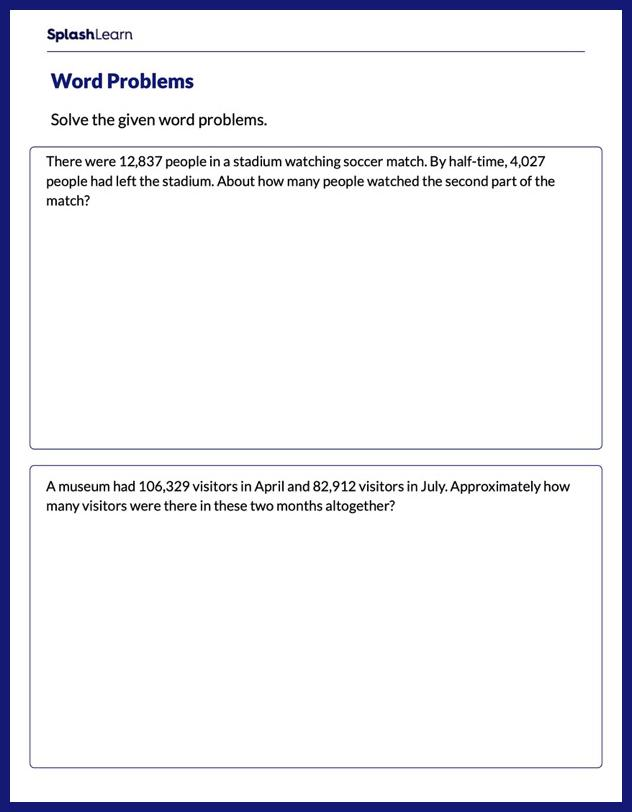# Word Problems on Estimating Sum or Difference Worksheet

Home > Word Problems on Estimating Sum or DifferenceStruggles with addition and subtraction can easily be overcome if students practice the concept in a fun and engaging way! Young learners will make connections between math and the real world as they solve a set of addition and subtraction word problems involving multi-operation scenarios. In these problems, they comprehend the scenarios and estimate the answer. Your student will develop the required confidence by solving a variety of problems on addition and subtraction.